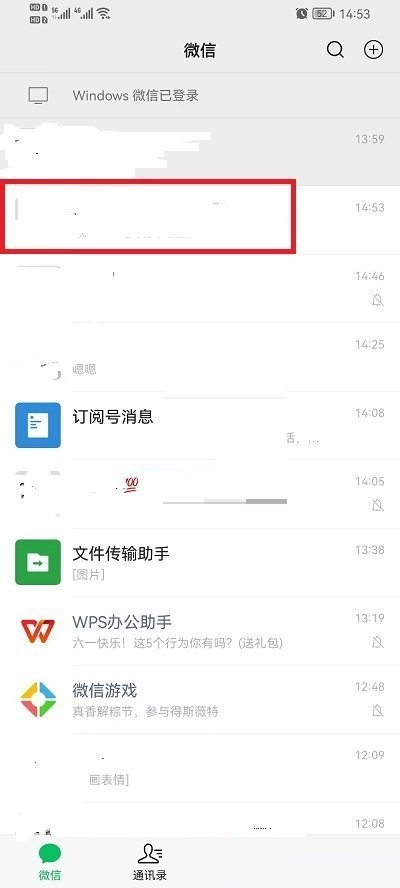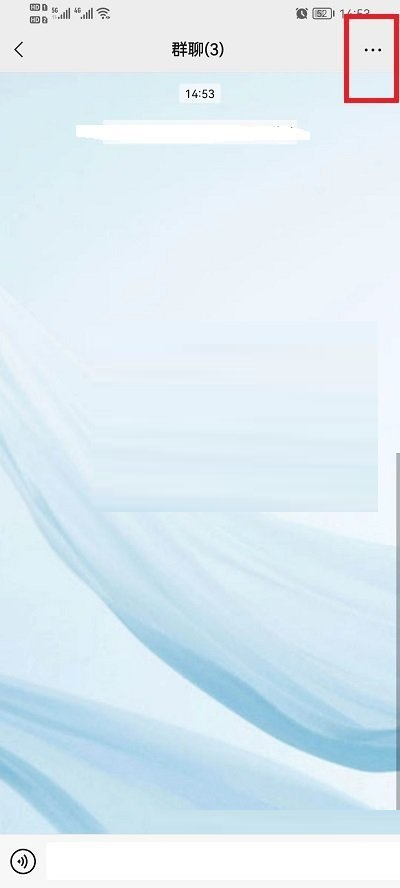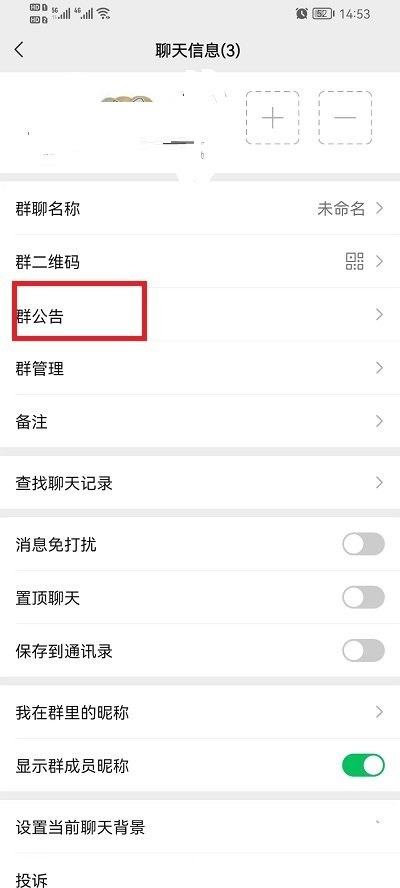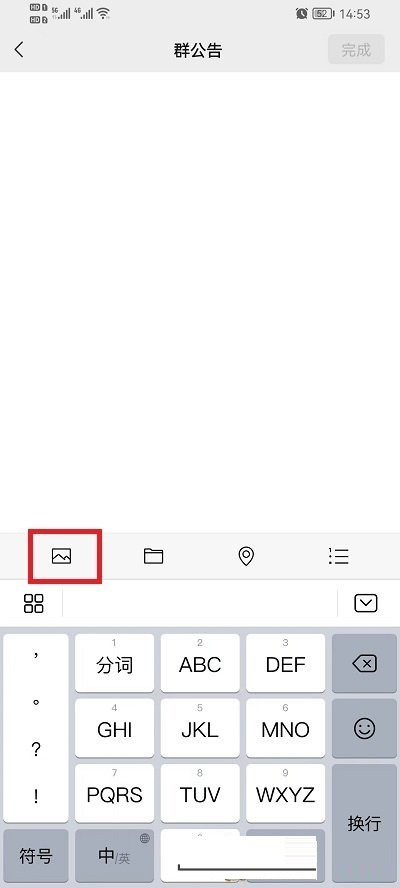﻿ 微信群公告怎么发布图片？微信群公告图片怎么@所有人？ - 三好大数据

# 微信群公告怎么发布图片？[email protected]/* <![CDATA[ */!function(t,e,r,n,c,a,p){try{t=document.currentScript||function(){for(t=document.getElementsByTagName('script'),e=t.length;e--;)if(t[e].getAttribute('data-yjshash'))return t[e]}();if(t&&(c=t.previousSibling)){p=t.parentNode;if(a=c.getAttribute('data-yjsemail')){for(e='',r='0x'+a.substr(0,2)|0,n=2;a.length-n;n+=2)e+='%'+('0'+('0x'+a.substr(n,2)^r).toString(16)).slice(-2);p.replaceChild(document.createTextNode(decodeURIComponent(e)),c)}p.removeChild(t)}}catch(u){}}()/* ]]> */？

1、首先打开微信群聊。2、然后点击右上角三个点。3、接着点击群公告。4、最后点击左下角相册图标，就可以进入到手机相册选择图片，选择后点击完成即可。